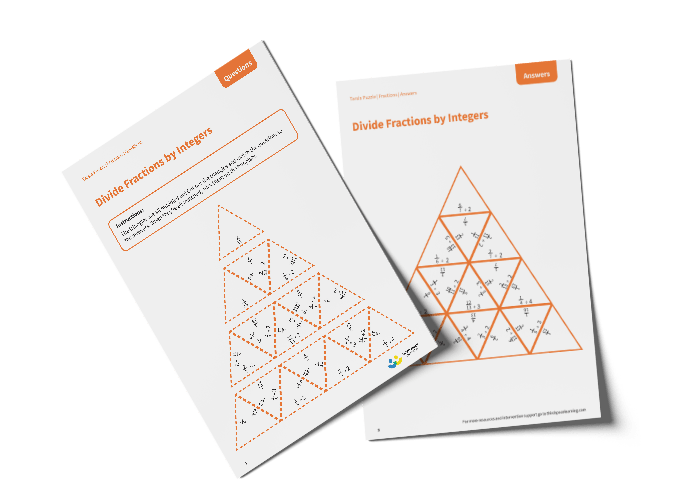# Tarsia Puzzle Divide Fractions by Integers (Year 6)

The triangles in the tarsia puzzle have all been mixed up. In this dividing fractions by integers tarsia puzzle, pupils will need to match the division question to the correct answer to create one large triangle.

This Year 6 divide fractions by integers tarsia puzzle worksheet contains:

• One question sheet
• One template sheet

Year 6

Fractions